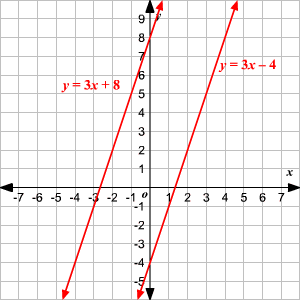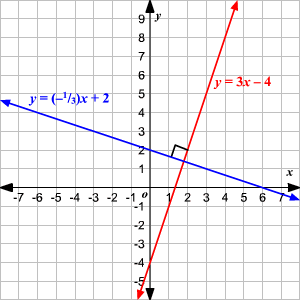# Parallel and Perpendicular Lines

Parallel lines are coplanar lines that never intersect.

In two dimensions, parallel lines have the same slope .

Example:

The two lines

$\begin{array}{l}y=3x+8\\ y=3x-4\end{array}$

are parallel, since they both have slope $3$ .Perpendicular lines are lines that intersect at right angles. If you multiply the slopes of two perpendicular lines, you get $-1$ .

Example:

The lines

$\begin{array}{l}y=-\frac{1}{3}x+2\\ y=3x-4\end{array}$

are perpendicular, since

$\left(-\frac{1}{3}\right)\left(3\right)=-1$Exception : A horizontal line and a vertical line are perpendicular, even though the product of their slopes $\left(0×\infty \right)$ is undefined.